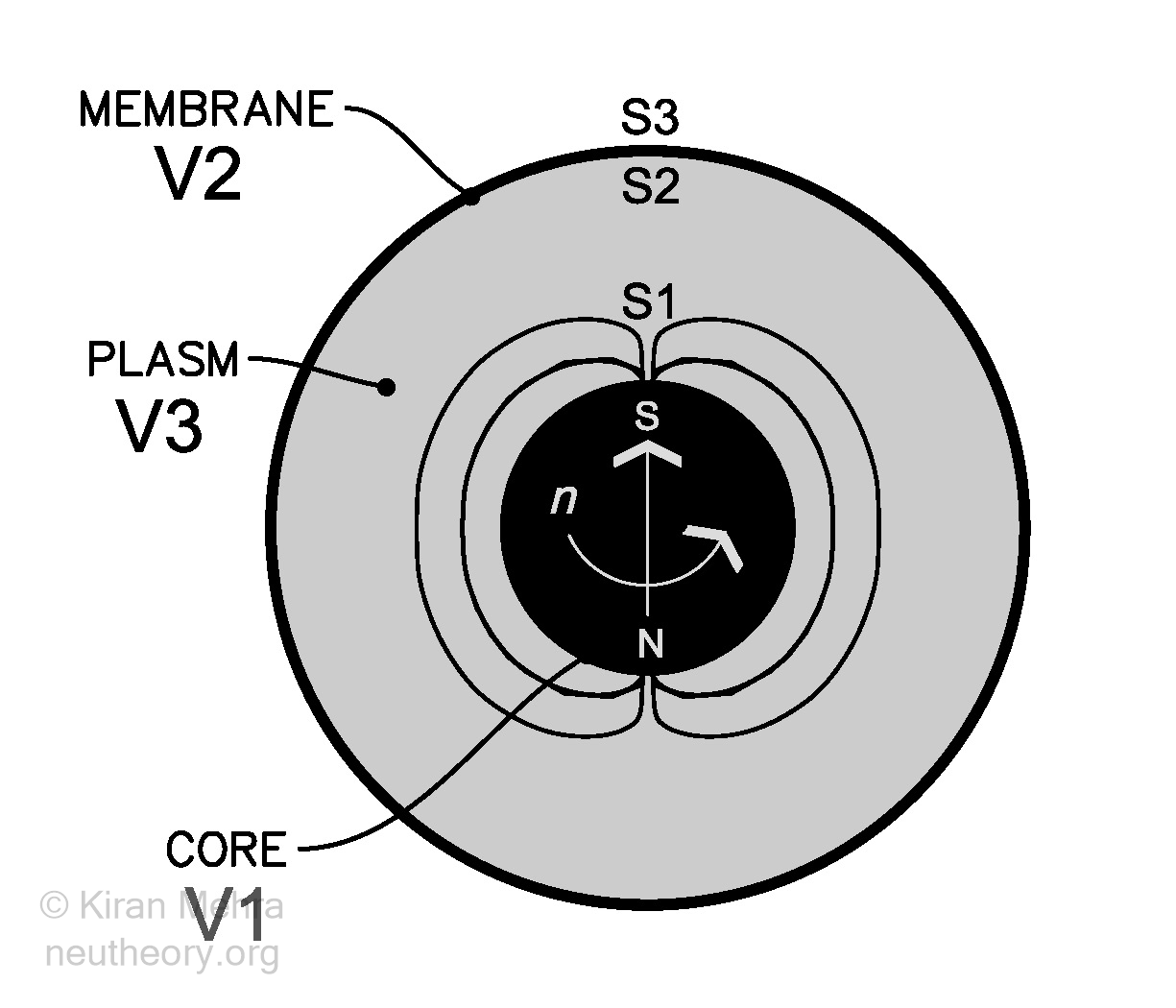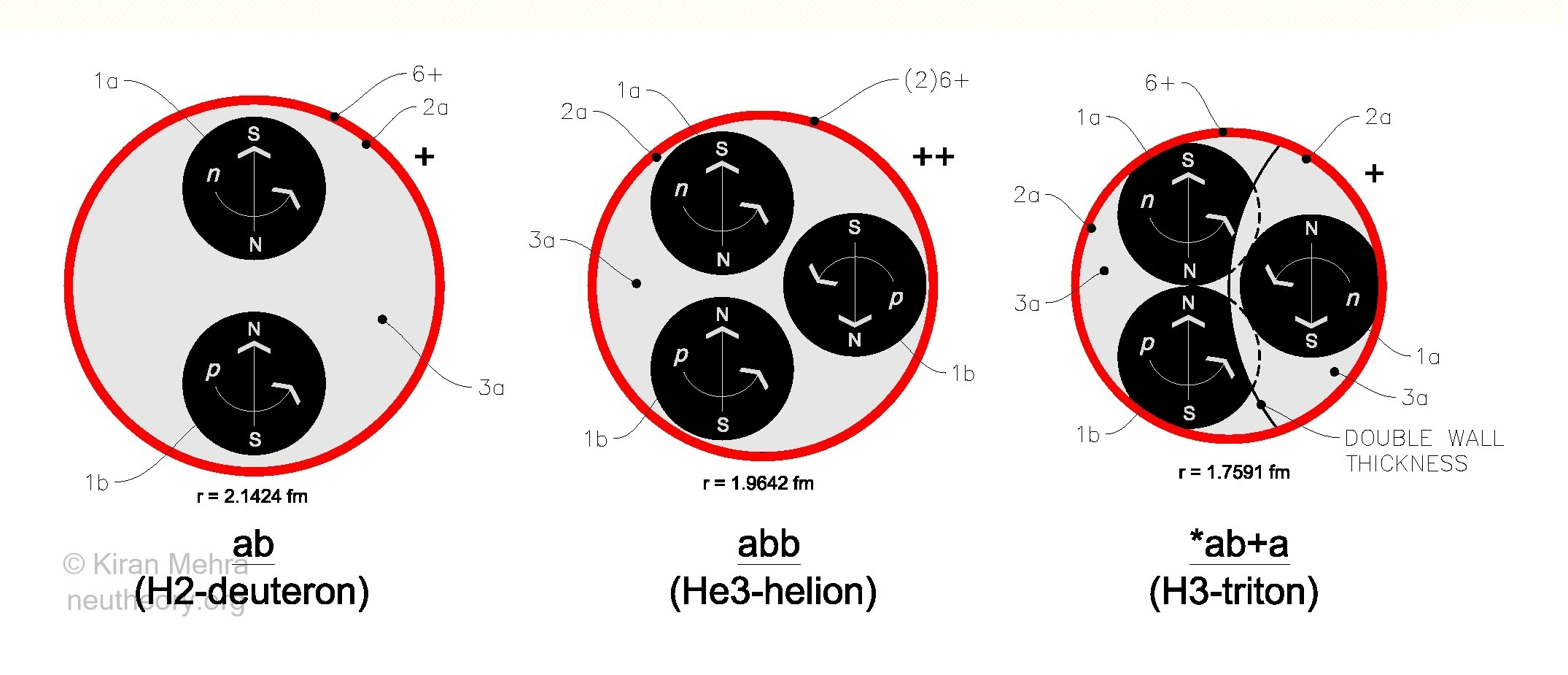NEU Theory

## NEU Theory

The Nature of Physical Reality

# Neu Mass & Charge Radii Table

of

### Introduction

The Neu Mass & Charge Radii Table calculates the nuclear physical properties of approximately 350 natural and selected radioactive isotopes based on a topological model that uses a hypothesized invariant 3 part neutron structure as a natural quantum unit of atomic matter. See Figure 1.Figure 1 – The Primal Prototype Object[/caption]

The currently accepted atomic mass unit (amu) is the Dalton unified atomic mass unit which is based on 1/12th of the mass of a C12 atom, and is calculated as approximately equal to 1.660 539 066 x 10-27 kg. With this NIST standard the relative mass of a neutron is equal to 1.008 664 915 amu.

Neu Theory in principle sets the neutron equal to one atomic matter unit. The Neu Theory amu is called the neu (neutron equivalent unit) and its measured value is approximately equal to 1.674 927 498 x 10-27 kg of mass. The neu is an absolute unit – a precise invariant quantity – numerically equal to exactly one quantum unit of matter in nature.

The neu is slightly larger than the C12 amu representing an increase in relative mass value of approximately 0.8665 %. The atomic masses of the isotopes in the table are then compared to the neu unit quantity with a corresponding decrease in relative mass value of approximately 0.8595 % as compared to the C12 value. The absolute neu value of C12 becomes 11.896 914 249 instead of 12.000 000 000. Column I in the table provides the relative C12 values as published by the National Institute of Science and Technology (updated September 21, 2016), Column J provides the equivalent calculated absolute neu mass value of selected isotopes.

It should be clearly emphasized that the actual measured mass of atoms in kilograms and the equivalent energy value in joules, electron volts, or any other unit, is not changed in the slightest by using a neutron amu. The numerically smaller quantum neu mass value of an isotope is exactly balanced by the numerically larger quantum neu mass unit giving us precisely the same quantity of mass expressed in kilograms, that we had before.

The reason an absolute neu can be useful, is that it allows mass and energy to be counted with the same numeric scale, and allows us in principle to estimate the ratio of matter to energy in the cosmos.

The table consists of two major parts:

• Columns A-Z – Neu Mass and other derived atomic values
• Columns AA-BL – Charge Radius derived values
All nuclides have an effective physical volume that is measured by their charge radius, a quantity that can be precisely measured, and is capable of providing a clear difference between the relative size of isotopes. The Neu Theory charge radii table uses values empirically determined and originally published in September 1999 by the International Nuclear Data Committee of the International Atomic Energy Agency and the adjusted values published in December 2012 by I. Angeli and K.P. Marinova. Some charge radii of some radioactive isotopes are not available. If these source values change or additional charge radii values become available, the table values will correspondingly adjust.

For simplicity, the charge radius is considered as defining the limiting spherical volume within which the neucleon cluster fits. In reality it is hypothesized, the charge shield  will uniformly cover the underling cluster, whatever its shape. The neucleon membrane walls do a "best fit", and the neuclonic plasms adjust their density (volume) as needed until a stable cluster configuration is reached.

### Nuclear Particles

The Neu theory nuclear model uses 5 of the 7 elementary particles of nature [1a][2a][3a][1b][6+]. These are the only physical forms that play an active role in all nuclear structure.

 tblcol form# elementary particle shape substance neu value volume density thickness structure spin/magnetism g-force O [1a] neutron core sphere *Type I matter 0.998623u fixed fixed (= r) homogenous ↑↓ = -1.000 g-rise P [2a] neutron membrane deformable shell *Type I matter 0.000544u fixed fixed varies homogenous none g-rise Q [3a] neutron plasm deformable shell **Type II matter 0.000833u varies varies varies homogenous none g-rise S [1b] captive proton core sphere *Type I matter ≤0.996255u varies fixed (= r) homogenous ↑↑ = (+ value varies with size) g-rise AD [6+] positive charge shell deformable shell split spin energy (½ 0.000833u) fixed fixed varies homogenous none g-fall

*Type I matter is at an absolute density of ~6.693 x 1017 kg/m3, and a specific volume of ~2.502 x 10-45 m3/neu.
**Type II matter varies in volume & density with nuclides.

These 5 particles make the three neucleons, which make all the nuclides that are listed as the elements and isotopes in the table. For each isotope the table generates fundamental properties at three subatomic levels:

• The 3rd or ordinary level is the measured and calculated physical properties of the isotope nucleus as a whole, i.e.,  neucleon makeup, total neu mass, net spin/magnetism, charge radius, nuclide volume, average density, nuclide g-rise, average electric charge shell thickness.
• The 2nd or primary level is the physical properties of the individual neucleon cells, i.e., cell neu mass, average volume , average density of the cells that make up the isotope.
• The 1st or elementary level (nature’s "bottom") are the individual elementary particles, i.e., the calculated physical properties of the individual cores, membranes, plasms and charge shells that make up the different elements and isotopes.

### Neucleon CellsFigure 2 – The Three Neucleons[/caption]

All atomic nuclides in nature are built of some combination of three primary cells called neucleons. See Figure 2. The size and structure of the 3 free neucleons is as noted and they are drawn to approximate relative scale. Neutron membrane thickness [2a] and charge shell thickness [6+] is symbolic and not to scale.

• The ab deuterons (Column F) are built from 5 elementary particles [1a][2a][3a][1b][6+]
• The abb helions (no column provided in the table) are built from 7 elementary particles [1a][2a][3a][1b][1b][6+][6+]
• The a neutrons (Column G) are built from 3 elementary particles [1a][2a][3a] and as they have no charge shell of their own, are only stable below some other nuclide charge shield. Hydrogen-3 with 1 deuteron + 1 neutron is shown (*ab+a).

In this model all stable nuclides and most radioactive nuclides are made from deuterons and neutrons only. The helion (He3) is stable as a free nuclide, however is unstable as a neucleon within a larger cluster, radioactively transforming (typically by positron emission) until a stable nuclide cluster is reached that contains only deuteron and neutron cells.

All nuclide clusters are held together below concentric layers of positive electric charge shells equal to the deuteron number. It should be noted that the model requires that there be no individual proton surfaces with an attached charge shell within a nuclide.

All protons within a nuclide are captive protons within neutron cells with their electric charge shells having migrated above the neutral neutron membrane surface(s). It is hypothesized by the model, that all nuclide mass loss (or gain) from radioactivity, comes from captive protons only. This reduction of proton mass is considered as the "binding mass" loss required to maintain nuclear stability, and in this model, is equivalent to twice the "binding energy" value of conventional science.

Neu Theory does not require a “strong” attractive nuclear force to hold the nucleus together. Uniform universal acceleration of the electric charge shield envelope creates g-fall, a compression force that acts in an opposite direction to nuclide cluster g-rise (AH). The reaction to g-rise pressure creates tension within the charge shield, and its thickness (AC) decreases. When equilibrium is reached between g-rise (AH) pressure and g-fall tension (in principle this value is equal and opposite to g-rise), a stable nuclear size and stable average charge shell thickness (AD) is maintained.

### Table Layout

ORIGINAL DATA SOURCE

Columns A, C, D, V, W, X, Y, Z – Handbook of Chemistry and Physics, 1988 edition, by CRC Press, Inc.: Wikipedia
Column I – Atomic Weights and Isotopic Compositions, National Institute of Science and Technology, September 2016
Column AA – Table of Experimental Nuclear Ground State Charge Radii: An Update, By I. Angeli and K.P. Marinova, December 2012

Column AB – Nuclear Isotope Database, EasySpin.org; (Pyykko and others)

### Neu Mass & Charge Radii Table

(click on image to go to table)